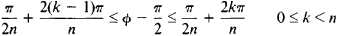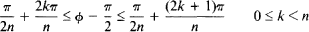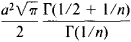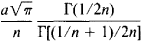# Sinusoidal Spiral

## sinusoidal spiral

[‚sī·nə′sȯid·əl ′spī·rəl]
(mathematics)
A plane curve whose equation in polar coordinates (r, θ) is r n = a n cos n θ, where a is a constant and n is a rational number.
McGraw-Hill Dictionary of Scientific & Technical Terms, 6E, Copyright © 2003 by The McGraw-Hill Companies, Inc.
The following article is from The Great Soviet Encyclopedia (1979). It might be outdated or ideologically biased.

## Sinusoidal Spiral

a curve whose equation in polar coordinates has the form

(*) rn = an sin nΦ

where n is a rational number. Special cases of a sinusoidal spiral include the circle, line, equilateral hyperbola, lemniscate, cardioid, and parabola, obtained from (*) by putting n = 1, − 1, -2, 2, 1/2, and - 1/2, respectively. If n = 0, then (*) becomes meaningless. Nevertheless, the logarithmic spiral can be regarded as a kind of limiting case corresponding to n = 0 that separates the sinusoidal spirals lying in a finite section of the plane from sinusoidal spirals with infinite branches.

The projection of the center of curvature at any point of a sinusoidal spiral on the radius vector of the point divides the radius vector in the ratio n : 1 (counting from the pole). If the radius vector of a sinusoidal spiral is made to rotate uniformly about the pole, the tangent also rotates uniformly about the point of tangency. For this reason, sinusoidal spirals are also called curves of proportional bending.

When n is a natural number, the sinusoidal spiral consists of n lobes enclosed within the anglesand tangent to the sides of the angle at the origin. The anglesdo not contain points of the sinusoidal spiral, other than the origin. If a regular n-gon P1, P2, • • •, Pn is inscribed in a circle of radius a2-1/n then a sinusoidal spiral is formed by the set of points for which the product of the distances to the points P1, P2, • • •, Pn is an/2. The area of one lobe of a sinusoidal spiral is equal toand the perimeter is equal towhere Γ(x) is the gamma function.

For natural n, the sinusoidal spiral has n axes of symmetry. If n = I/q, the curve is symmetric about the polar axis, and each of its halves is in the form of a spiral that begins at the point r = a, Φ = π/2, and passes through the pole after a revolution of /2. When n = p/q, the sinusoidal spiral is an algebraic curve (seeALGEBRAIC GEOMETRY) with p axes of symmetry forming angles of 2πqk/p, 0 ≤ k < p, with the vertical axis.

The study of sinusoidal spirals with negative n can be reduced to the case of positive n by means of an inversion. Sinusoidal spirals are encountered in certain problems in, for example, mechanics and geodesy. [23–1296–]